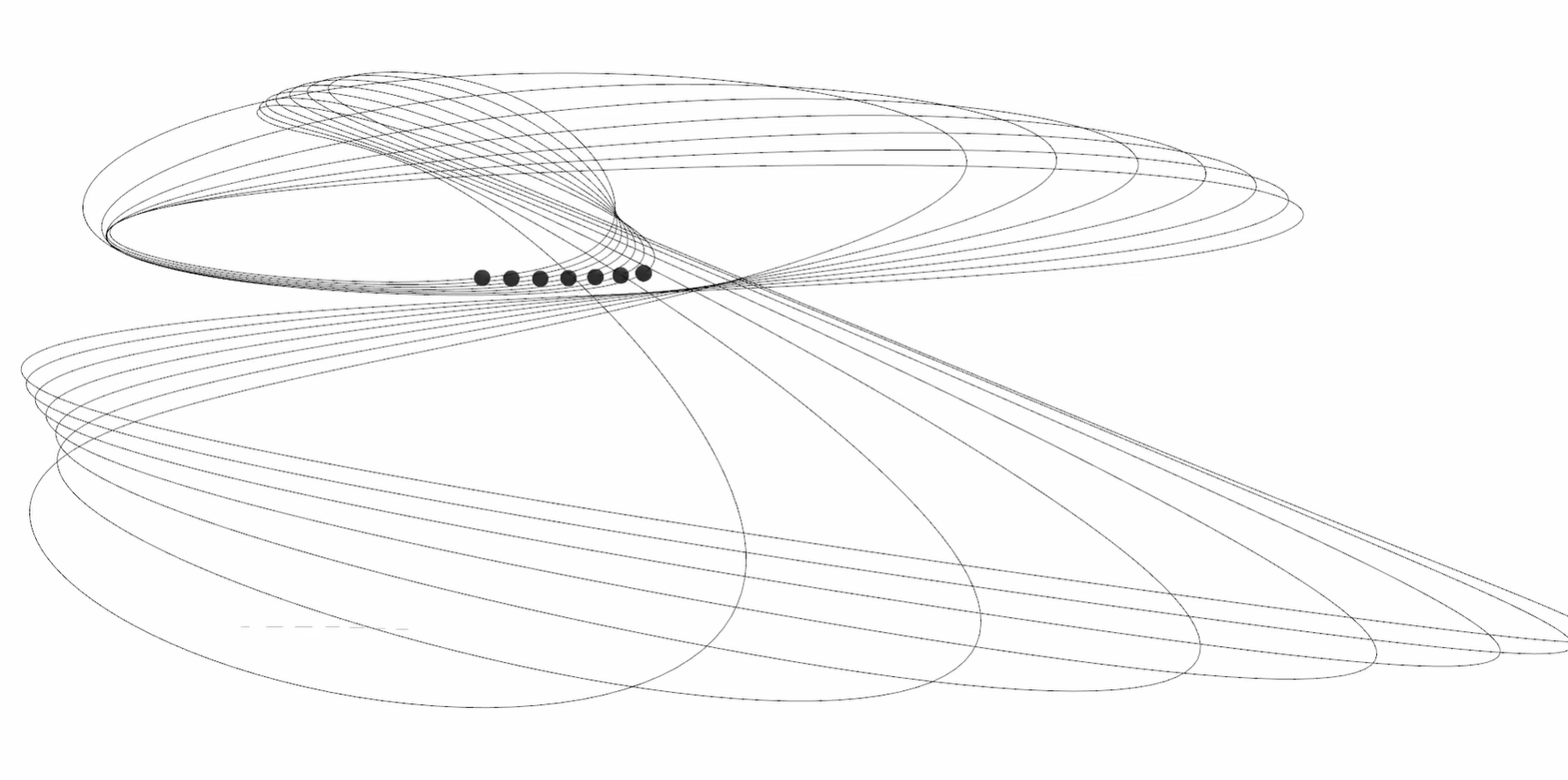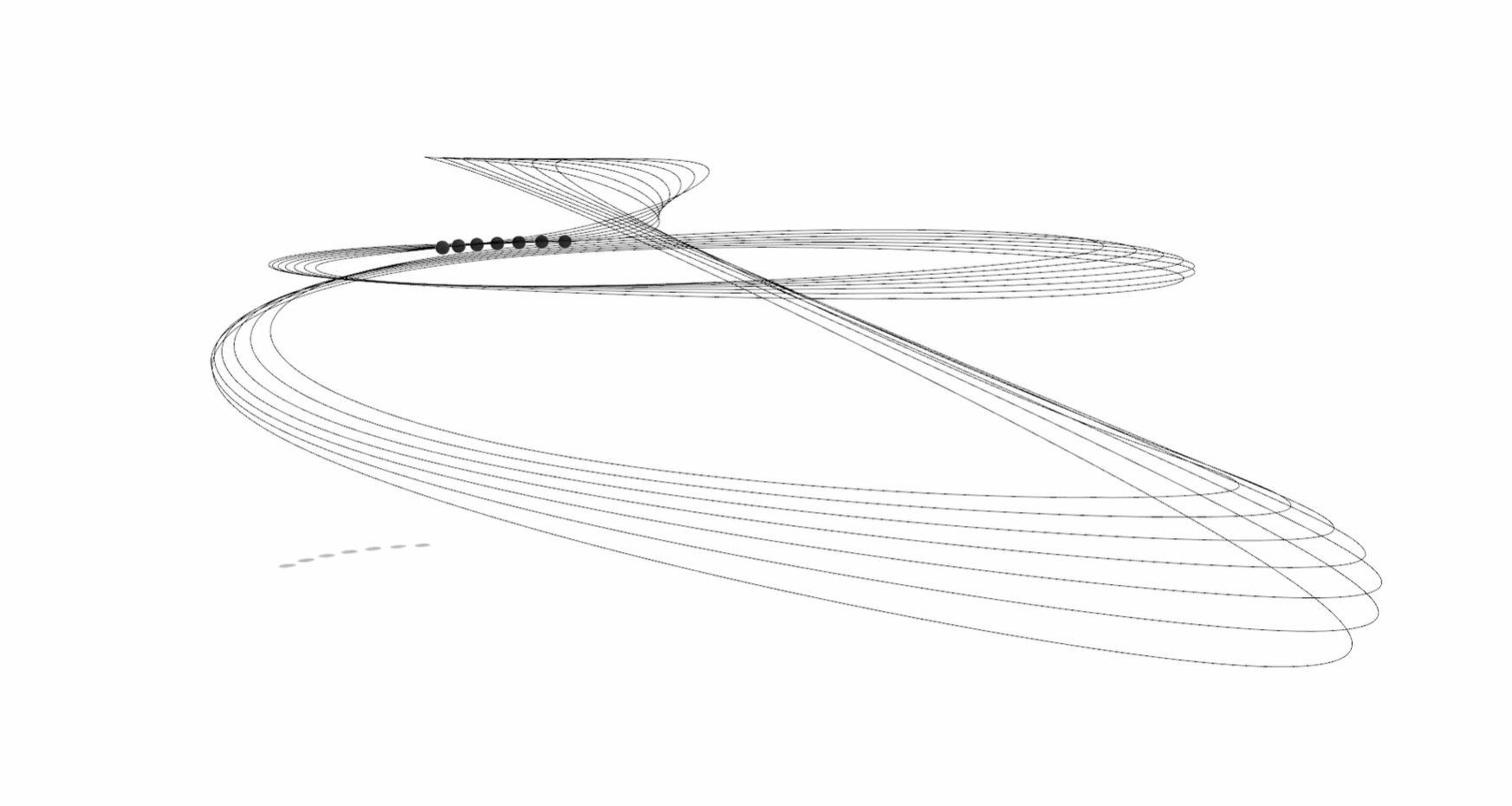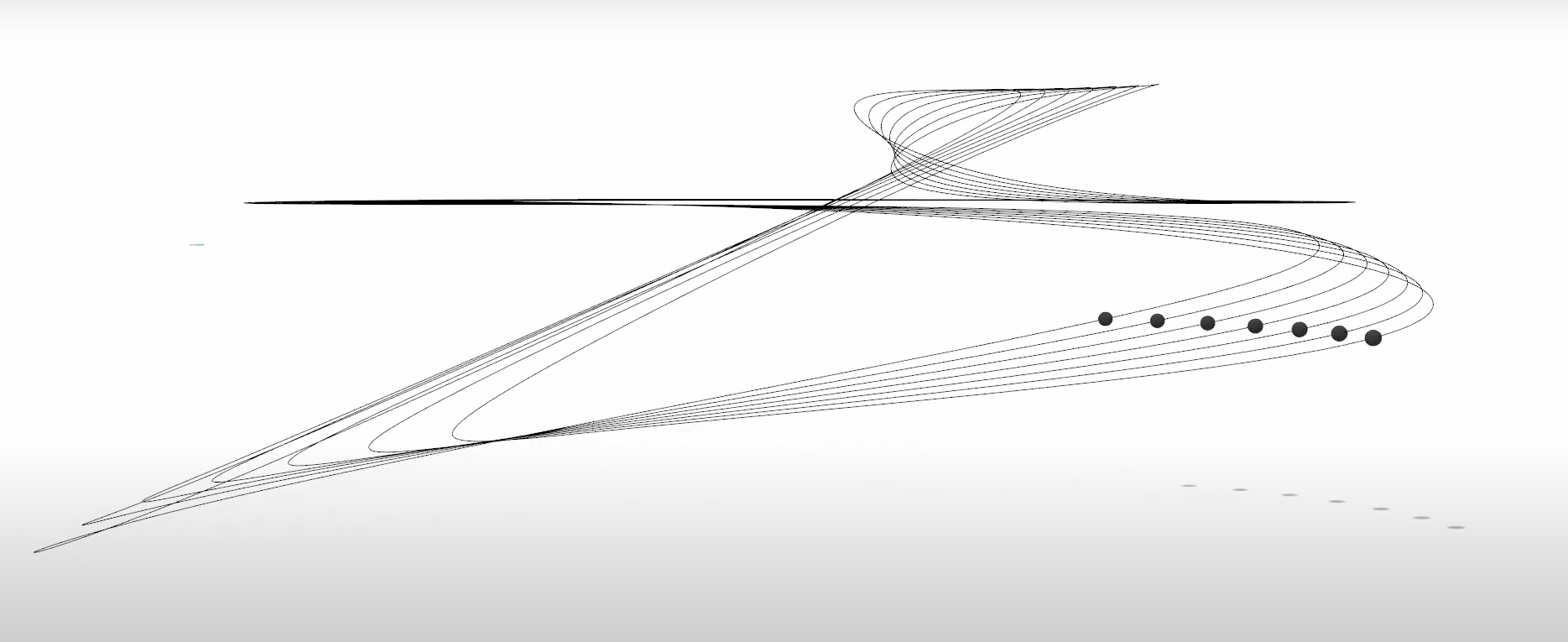### Frame by frame screenshot capture for Rhino3D Python script

This is a concept test for creating a frame by frame animation using Rhino and Python. It’s definitely not the fastest way to create an animation but it may be a useful tool to visualize evolving data through time and have a better understanding of some optimization algorithms strategies within a script.

I was pleased with the time it took to create an individual frame. I will try to further implement it on a rigid body optimization software using a genetic algorithm that I have been working for a while now.

Here are the videos I made to test the concept as well as the Python script.## Videos

The videos were merged using Resolve.

## Python script

Rhino Python standard imports.

``````import rhinoscriptsyntax as rs
import os
``````

The Timelapse class.

``````class Timelapse():

def __init__(self,folder='',size=(1920,1080),prefix='',format='png'):
self.frame = 0
self.folder = folder
self.size = size
self.prefix = prefix
self.format = format

def screenshot(self):
save_path = os.path.join(self.folder,'%s_%s.%s'%(self.prefix,self.frame,self.format))
rs.CreatePreviewImage(save_path, view=None, size=self.size, flags=2, wireframe=False)
self.frame += 1
return self.frame
``````

The Sphere class.

``````class Sphere():

self.location = location if location else path
self.color = self.set_color(color)
self.material = None
self.set_material(color)
self.path = path
self.frame = 0

def move(self,position):
rs.MoveObject(self.object,tuple([sum(x) for x in zip(position,(-n for n in self.location))]))
self.location = position
return position

self.frame += 1
return self.move(self.path[self.frame])

def set_color(self,color):
rs.ObjectColor(self.object,color)
self.color = color
return color

def set_material(self,color):
if rs.ObjectMaterialIndex(self.object) == -1:
rs.MaterialColor(self.material,color)
self.color = color
return color
``````

Tests the animation.

``````def run_test():
# Set time lapse length
max_frames = 330
# Sets folder to store images and creates a time lapse object
folder = rs.BrowseForFolder(message='Select folder to store frames')
timelapse = Timelapse(folder=folder,size=(1920,1080),prefix='test',format='png')
# Creates a point array from base curves to specify a motion path for the spheres to follow
curves = rs.GetObjects("Select curves for path vector", rs.filter.curve,True,True)
point_paths = [rs.DivideCurve(curve,max_frames+1) for curve in curves]
# Creates the sphere objects
spheres = [Sphere(20,None,(50,50,50),path) for path in point_paths]
# Run until frame rate hits max frames
while timelapse.frame < max_frames:
for sphere in spheres:
``````if __name__=="__main__":